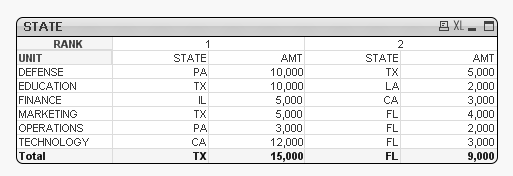# QlikView App Dev

Discussion Board for collaboration related to QlikView App Development.

Announcements
cancel
Showing results for
Did you mean:Creator II

## How to get TOTAL of a Ranked Column

Hello,

I've a STATE table as listed below. Now, I need a total row that displays a state with the highest amount (sum of all the amounts for that state irrespective of the UNIT). Any ideas?1 Solution

Accepted SolutionsMVP

Not the best way to do this, but can't think of a better way

STATE

If(Dimensionality() = 0,

Pick(Aggr(Rank(Sum({< DATE = {'201806'}>} AMOUNT)), UNIT, STATE),

FirstSortedValue(DISTINCT STATE, -Aggr(Sum(TOTAL <STATE> {< DATE = {'201806'}>} If(Aggr(Rank(Sum({< DATE = {'201806'}>} AMOUNT)), UNIT, STATE) = 1, AMOUNT)), UNIT, STATE)),

FirstSortedValue(DISTINCT STATE, -Aggr(Sum(TOTAL <STATE> {< DATE = {'201806'}>} If(Aggr(Rank(Sum({< DATE = {'201806'}>} AMOUNT)), UNIT, STATE) = 2, AMOUNT)), UNIT, STATE))),

AGGR(IF(RANK(SUM({< DATE = {'201806'} >} AMOUNT)) <= 2 , STATE, 'All Other'), UNIT, STATE))

AMT

If(Dimensionality() = 0,

Pick(Aggr(Rank(Sum({< DATE = {'201806'}>} AMOUNT)), UNIT, STATE),

Max(Aggr(Sum(TOTAL <STATE> {< DATE = {'201806'}>} If(Aggr(Rank(Sum({< DATE = {'201806'}>} AMOUNT)), UNIT, STATE) = 1, AMOUNT)), UNIT, STATE)),

Max(Aggr(Sum(TOTAL <STATE> {< DATE = {'201806'}>} If(Aggr(Rank(Sum({< DATE = {'201806'}>} AMOUNT)), UNIT, STATE) = 2, AMOUNT)), UNIT, STATE))),

Sum({<DATE = {'201806'}>} AMOUNT) + Sum({1}0))4 RepliesMVP

Not the best way to do this, but can't think of a better way

STATE

If(Dimensionality() = 0,

Pick(Aggr(Rank(Sum({< DATE = {'201806'}>} AMOUNT)), UNIT, STATE),

FirstSortedValue(DISTINCT STATE, -Aggr(Sum(TOTAL <STATE> {< DATE = {'201806'}>} If(Aggr(Rank(Sum({< DATE = {'201806'}>} AMOUNT)), UNIT, STATE) = 1, AMOUNT)), UNIT, STATE)),

FirstSortedValue(DISTINCT STATE, -Aggr(Sum(TOTAL <STATE> {< DATE = {'201806'}>} If(Aggr(Rank(Sum({< DATE = {'201806'}>} AMOUNT)), UNIT, STATE) = 2, AMOUNT)), UNIT, STATE))),

AGGR(IF(RANK(SUM({< DATE = {'201806'} >} AMOUNT)) <= 2 , STATE, 'All Other'), UNIT, STATE))

AMT

If(Dimensionality() = 0,

Pick(Aggr(Rank(Sum({< DATE = {'201806'}>} AMOUNT)), UNIT, STATE),

Max(Aggr(Sum(TOTAL <STATE> {< DATE = {'201806'}>} If(Aggr(Rank(Sum({< DATE = {'201806'}>} AMOUNT)), UNIT, STATE) = 1, AMOUNT)), UNIT, STATE)),

Max(Aggr(Sum(TOTAL <STATE> {< DATE = {'201806'}>} If(Aggr(Rank(Sum({< DATE = {'201806'}>} AMOUNT)), UNIT, STATE) = 2, AMOUNT)), UNIT, STATE))),

Sum({<DATE = {'201806'}>} AMOUNT) + Sum({1}0))Creator II
Author

Hi Sunny, this works although there might be alternate ways.

Question: If I need to the Total for TX and FL and display in a separate text box - how can we do that?

For instance, TX = 15,000 & FL = 9,000. Requirement is to display this as "Other" - 24,000. So I was thinking to use a text box for the sum.MVP

For Rank 1

=Max(Aggr(Sum(TOTAL <STATE> {< DATE = {'201806'}>} If(Aggr(Rank(Sum({< DATE = {'201806'}>} AMOUNT)), UNIT, STATE) = 1, AMOUNT)), UNIT, STATE))

For Rank 2

=Max(Aggr(Sum(TOTAL <STATE> {< DATE = {'201806'}>} If(Aggr(Rank(Sum({< DATE = {'201806'}>} AMOUNT)), UNIT, STATE) = 2, AMOUNT)), UNIT, STATE))Creator II
Author

Ahh, got it. You are awesome. Thank you very much!!!